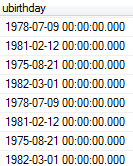select * from txl3 where ubirthday like '197[0-9]%'

3个回答

SELECT * from txl3 WHERE convert(varchar(40),ubirthday) LIKE '197[0-9]%'

SELECT * from txl3 WHERE convert(varchar(40),ubirthday) >= '1970' and convert(varchar(40),ubirthday) < '1980'

select * from txl3 where left(convert(varchar(40),ubirthday),2,4)='70'